Courses

# Previous Year Questions - Real Numbers Class 10 Notes | EduRev

## Class 10 : Previous Year Questions - Real Numbers Class 10 Notes | EduRev

The document Previous Year Questions - Real Numbers Class 10 Notes | EduRev is a part of the Class 10 Course Mathematics (Maths) Class 10.
All you need of Class 10 at this link: Class 10

Q.1. Find a rational number between √2 and √3.    [Delhi 2019]
Ans: Let p be rational number between √2 and √3
∴ √2 < p < √3
On squaring throughout, we have
2 < p2 < 3
The perfect squares which lie between 2 and 3 are 2 < 2.25 < 2.56 < 2.89 < 3.
Taking square root throughout
The rational numbers between √2 and √3 are 1.5, 1.6 1.7 and more.

Q.2. Prove that √2 is an irrational number.    [Delhi 2019]
Ans:  Let √2 = a/b where a and b are co prime integer, b ≠ 0.
Squaring both sides, we get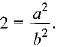Multiplying with b on both sides, we get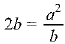Multiplying with b on both sides, we get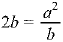LHS = 2 x b = Integer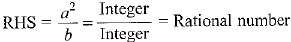∴ LHS ≠ RHS
∴ Our supposition is wrong.
⇒ √2 is irrational.

Q.3. Prove that 2 + 5 √3 is an irrational number, given that √3 is an irrational number.    [CBSE, Allahabad 2019]
Ans: Let 2 + 5 √3 be a rational number. (p and q are co-prime positive integers, q ≠ 0)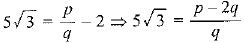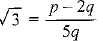which is a contradiction because √3 is an irrational number and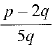is  a rational.

Q.4. The HCF of two numbers a and b is 5 and their LCM is 200. Find the product ab.    [CBSE 20 19 (30/5/2)]
Ans: We know that
Product of two numbers = product of their LCM and HCF.
ab = 200 x 5
= 1,000

Q.5. A number N when divided by 14 gives the remainder 5. What is the remainder when the same number is divided by 7?    [CBSE 2019 (30/2/1)]
Ans: 5, because 14 is multiple of 7.

Q.6. Find after how many places the decimal form o f the number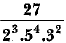will terminate.    [CBSE 2019 (30/3/3)
Ans: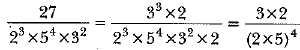So, the decimal form will end after four decimal places.

Q.7. Express 429 as product of its prime factors.    [CBSE 2019 (30/3/3)]
Ans: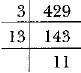∴ 429 = 3 x 13 x 11

Q.8. Find the HCF of 612 and 1314 using prime factorisation.    [CBSE 2019 (30/5/3)]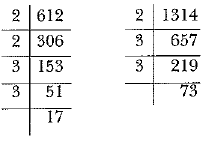Ans: 612 = 2 x 2 x 3 x 3 x 17
1314 = 2 x 3 x 3 x 73
HCF = 2 x 3 x 3 = 18

Q.9. Find the HCF o f 1260 and 7344 using Euclid’s algorithm.    [NCERT, CBSE 2019 (30/1/1)]
OR
Use Euclid’s division algorithm to find the HCF o f 255 and 867.    [CBSE 2019 (30/4/1)]
Ans:
Since 7344 > 1260 and from Euclid’s algorithm
a = bq + r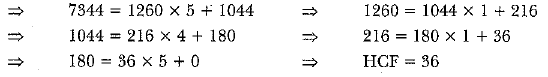OR
Solution is similar as above.
Only values are changed

Q.10. Write a rational number between √2 and √3.    [CBSE 2019 (30/1/2)]
Ans:
A rational number between √2 and √3 is √2.89 = 1.7 = 17/10.

Q.11. If HCF of 65 and 117 is expressible in the form 65n - 117, find the value of n.    [CBSE 2019 (30/3/3)]
Ans:
HCF (65, 117) is given by
65 = 5 x 13
117 = 13 x 3 x 3
⇒ HCF =13
According to question,
65n - 117 = 13
65n = 130
n = 2

Q.12. Write the smallest number which is divisible by both 306 and 657.    [CBSE 2019 (30/2/1)]
Ans:
Here, to find the required smallest number we will find LCM of 306 and 657.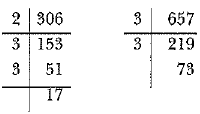306 = 2 x 32 x 17
657 = 32 x 73
LCM - 2 X 32 x 17 x 73 = 22338

Q.13. Show that any positive odd integer is of the form 6q + 1 or 6q + 3 or 6q + 5 where q is some integer.
[NCERT]
OR
Show that any positive odd integer is of the form 6 m + 1 or 6 m + 3 or 6 m + 5, where m is some integer.    CBSE 2019(30/5/2)]
Ans: Let a be any positive odd integer and b = 6. Then, by Euclid's algorithm, a = 6q + r, for some integer q > 0 and 0 < r < 6.
i.e., the possible remainders are 0, 1, 2, 3, 4, 5.
Thus, a can be of the form 6q or 6q + 1 or 6q + 2 or 6q + 3 or 6q + 4 or 6q + 5, where q is some quotient.
Since a is odd integer, so a cannot be of the form 6q or x 6q + 2 or 6q + 4 (since they are even).
Thus, a is of the form 6q + 1 or 6q + 3 or 6q + 5, where q is some integer.
Hence, any odd positive integer is of the form 6q + 1 or 6q + 3 or 6q + 5, where q is some integer.
OR
Let a be any positive odd integer and b = 6. Then, by Euclid’s algorithm, a = 6m + r, for some integer m > 0 and 0 < r < 6.
i.e., the possible remainders are 0, 1, 2, 3, 4, 5.
Thus, a can be of the form 6m, or 6m + 1 or 6m + 2 or 6m + 3 or 6m + 4 or 6m + 5, where m is some quotient.
Since a is odd integer, so a cannot be of the form 6m or 6m + 2 or 6m + 4 (since they are even). Thus, a is of the form 6m + 1, 6m + 3 or 6m + 5, where m is some integer.
Hence, any odd positive integer is of the form 6m + 1 or 6m + 3 or 6m + 5, where m is some integer.

Q.14. Find HCF and LCM of 404 and 96 and verify that HCF x LCM = Product of the two given numbers.    [CBSE 2018]
Ans: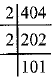HCF of 404 and 9
404 = 2 x 2 x 101
96 = 2 x 2 x 2 x 2 x 2 x 3
Common factor = 2 x 2 = 4
∴ HCF = 4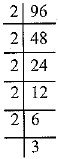LCM of 404 and 96
= 2 x 2 x 101 x 2x 2x 2 x 3 = 9696
Verification:
HCF x LCM = 4 x 9696 = 38784
Product o f two numbers = 404 x 96 = 38784
Clearly, HCF x LCM = Product of two numbers
Hence verified

Q.15. Write whether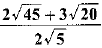on simplification gives an irrational or a rational number.    [CBSE 2018 (C)]
Ans: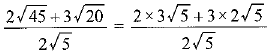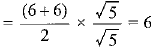(rational number)

Q.16. What is the HCF of smallest prime number and the smallest composite number?    [CBSE 2018]
Ans:
The smallest prime number = 2
The smallest composite number = 4
∴ HCF of 2 and 4 = 2

Q.17. Given that √2 is irrational, prove that (5 + 3 √2 ) is an irrational number    [CBSE 2018]
Ans:
Let 5 + 3 √2 = p/q  be a rational number, wherep and q q have no common factor other than 1 and q ≠ 0.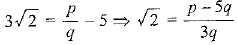For any values of p and q(q ≠ 0),  RHS =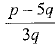is rational, but LHS = √2 is s irrational.
This contradicts the fact. So, our assumption is wrong.
∴ 5 + 3 √2  is an irrational number.

Q.18. Given that √3 is an irrational number, prove that (2 + √3 ) is an irrational number.    [CBSE 2018 (C)]
Ans: Let (2 + √3 is not an irrational.
∴ it can be written in the form a/b, where b ≠ 0 and a and b are coprime.
So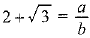Subtracting 2 from both sides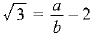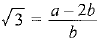This contradicts.
LHS is an irrational number because 3 is an irrational number (given) and RHS is an rational number.
So, LHS is equal to RHS not possible.
Hence, our assumption is wrong.
∴ (2 + √3 is an irrational number.

Q.19. What is the HCF of the smallest composite number and the smallest prime number?    [CBSE 2018]
Ans: Smallest composite number = 4
Smallest prime number = 2
So, HCF (4, 2) = 2

Q.1. Prove that √5 is an irrational number. [CBSE 2019 (30/5/1)]
Ans: Let us assume, to the contrary, that Vs is a rational number.
Then, there exist co-prime positive integers a and b such that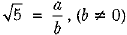So, a = √5 b
Squaring both sides, we have
a2 = 5b2   ......(i)
⇒ 5 divides a⇒ 5 divides a
So, we can write
a = 5c (where c is any integer)
Putting the value of a = 5c in (i), we have
25c2 = 5b2 => 5c2 = b
It means 5 divides b2 and so 5 divides b.
So, 5 is a common factor of both a and b which is a contradiction.
So, our assumption that √5  is a rational number is wrong.
Hence, we conclude that √5  is an irrational number.

Offer running on EduRev: Apply code STAYHOME200 to get INR 200 off on our premium plan EduRev Infinity!

## Mathematics (Maths) Class 10

84 videos|319 docs|103 tests

,

,

,

,

,

,

,

,

,

,

,

,

,

,

,

,

,

,

,

,

,

;